Pandas数据载入与预处理（详细的数据Python处理方法）黎明之道100 0 0

# Pandas数据载入与预处理

NumPy常用的导入格式：import pandas as pd

## 一、数据载入

txt文件：是Windows操作系统上附带的一种文本格式，文件以.txt为后缀。

CSV文件：是Comma-Separated Values的缩写，用半角逗号（’,’）作为字段值的分隔符。

pandas.read_table(filepath_or_buffer, sep=’\t’, header=’infer’, names=None, index_col=None, dtype=None, engine=None, nrows=None)

pandas.read_csv(filepath_or_buffer, sep=’，’, header=’infer’, names=None, index_col=None, dtype=None, engine=None, nrows=None)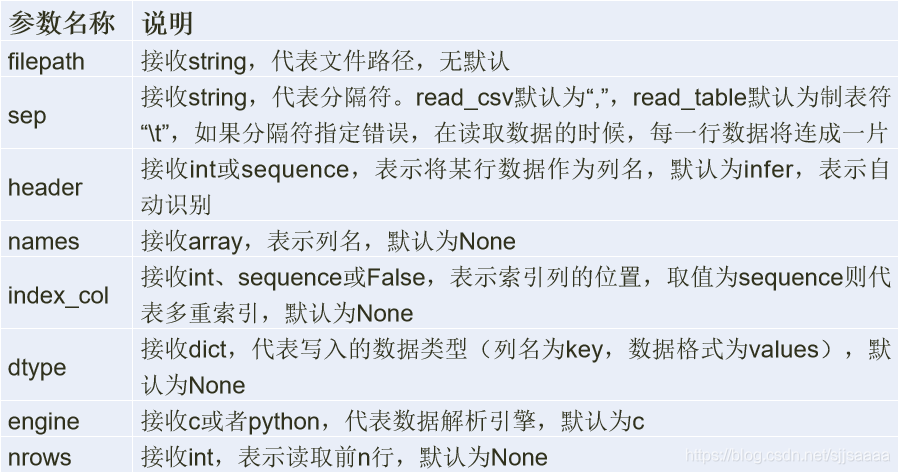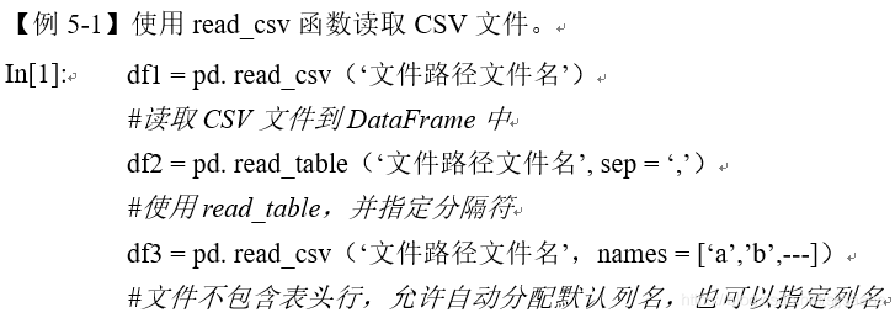DataFrame.to_csv(path_or_buf = None, sep = ’,’, na_rep, columns=None, header=True, index=True, index_label=None, mode=’w’, encoding=None)

Excel文件的读取

Pandas提供了read_excel函数读取“xls”和“xlsx”两种excel文件，其格式为：
pandas.read_excel(io, sheetname, header=0, index_col=None, names=None, dtype)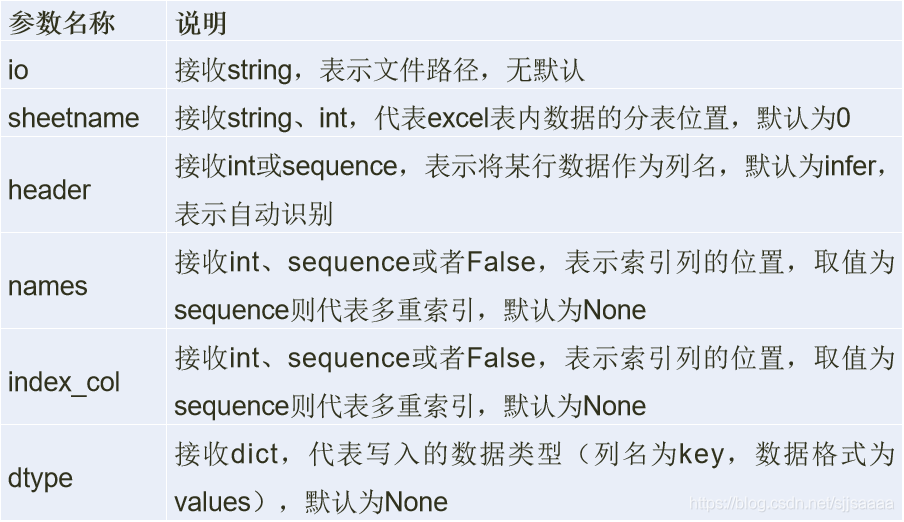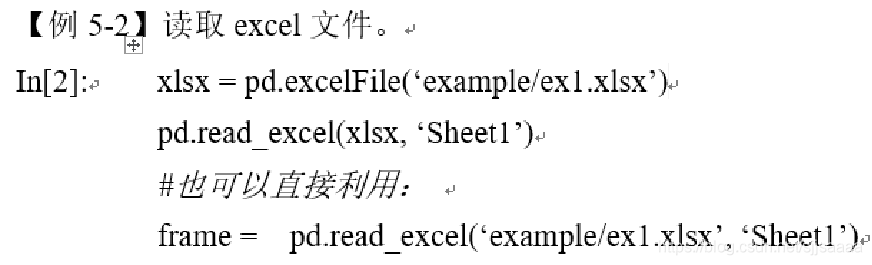Excel文件的存储

DataFrame.to_excel(excel_writer=None, sheetname=None’, na_rep=”, header=True, index=True, index_label=None, mode=’w’, encoding=None)

to_csv方法的常用参数基本一致，区别之处在于指定存储文件的文件路径参数excel_writer，增加了一个sheetnames参数，用来指定存储的Excel sheet的名称，默认为sheet1。

## 2.数据合并

merge数据合并

merge函数是通过一个或多个键将两个DataFrame按行合并起来，与SQL中的 join 用法类似，Pandas中的数据合并merge( )函数格式如下：
merge(left, right, how='inner', on=None, left_on=None, right_on=None, left_index=False, right_index=False, sort=False, suffixes=('_x', '_y'), copy=True, indicator=False, validate=None)

merge方法主要参数及说明：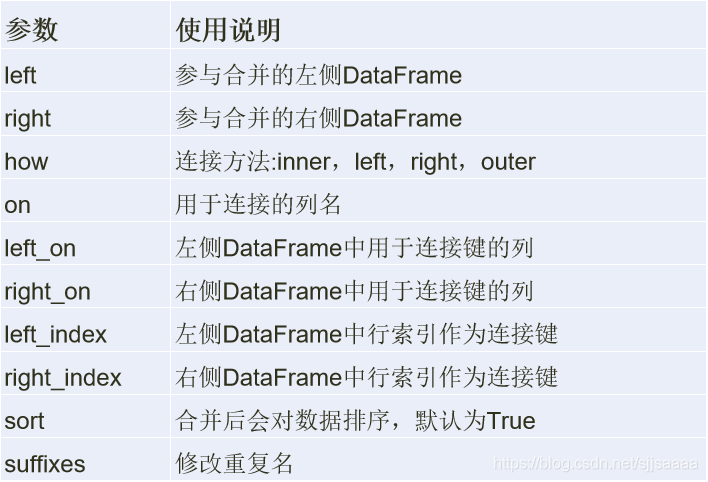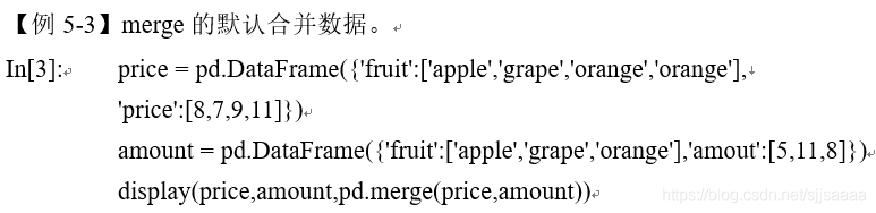concat数据连接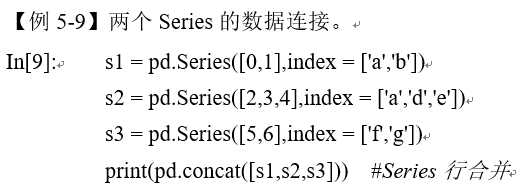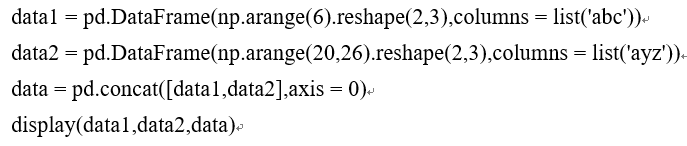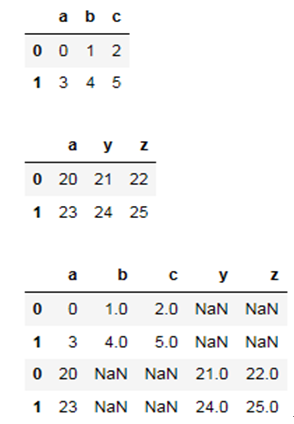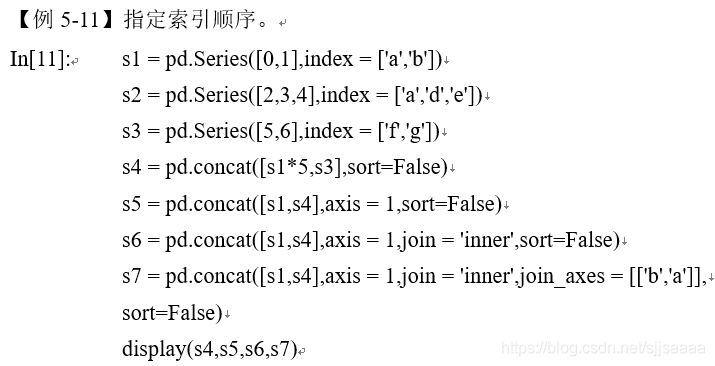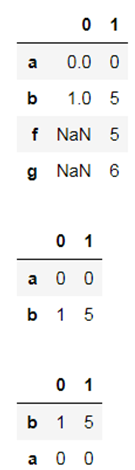combine_first合并数据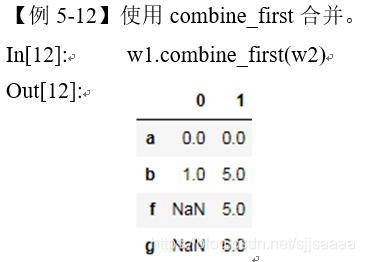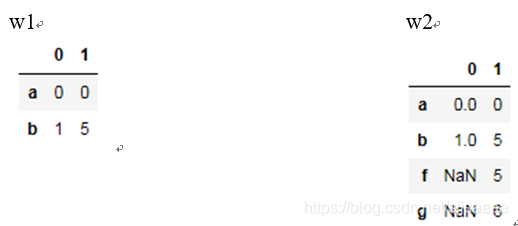## 3.数据清洗

isnull()函数可以直接判断某列中的哪个数据为NaN，利用isnull().sum()可以统计缺失值的缺失数目。

string_data = pd.Series(['aardvark', 'artichoke', np.nan, 'avocado'])
print(string_data)
string_data.isnull()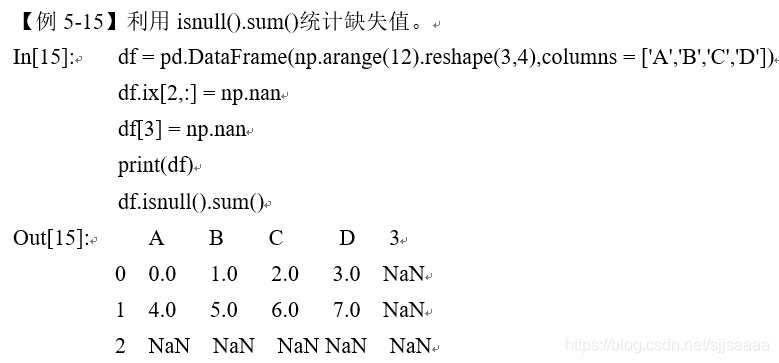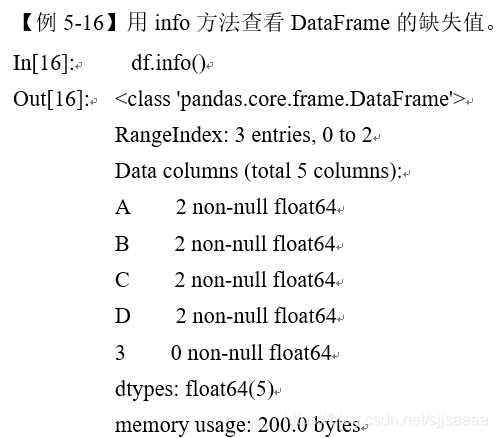dropna方法的格式：
dropna(axis=0, how=‘any’, thresh=None, subset=None, inplace=False)

dropna的参数及其使用说明：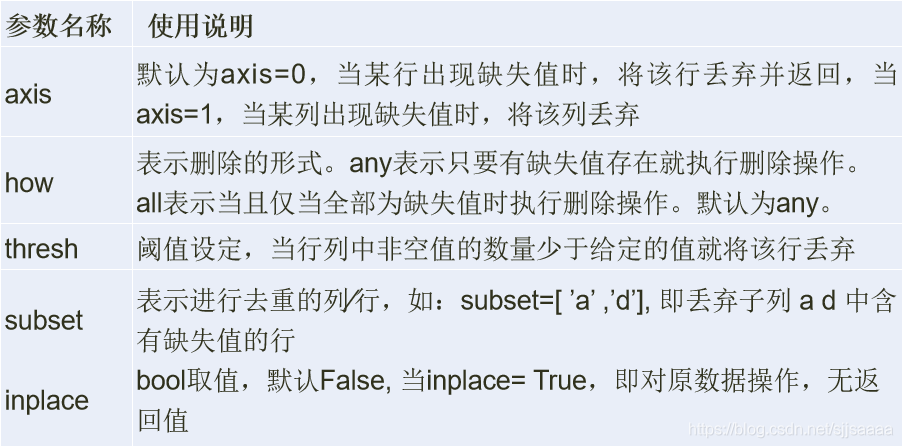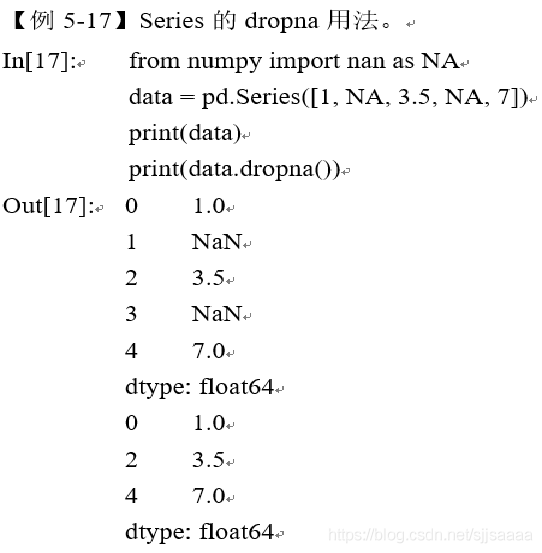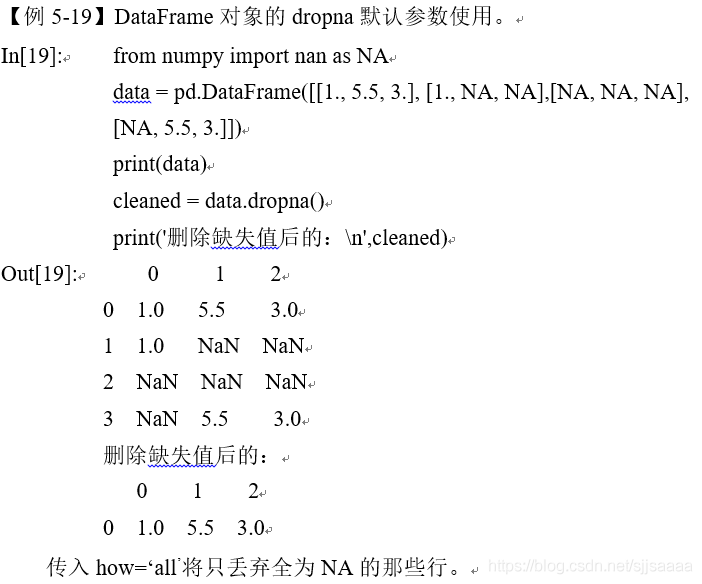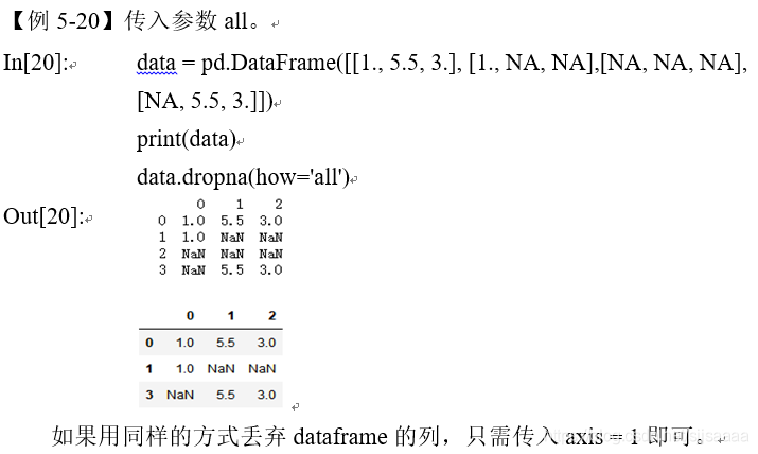Pandas库中提供了缺失值替换的方法fillna，格式如下：DataFrame.fillna(value=None, method=None, axis=None, inplace=False, limit=None)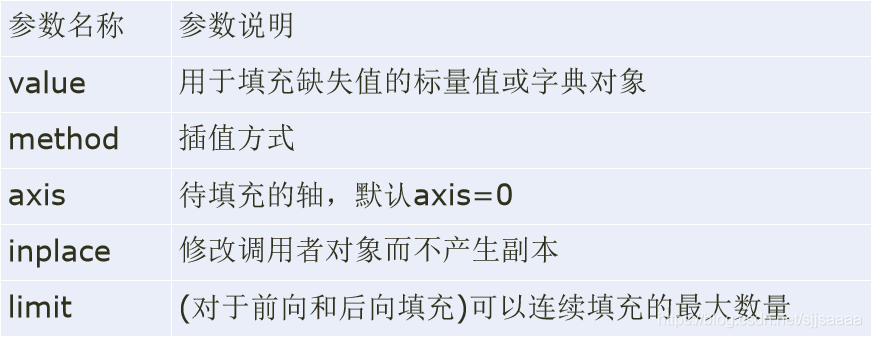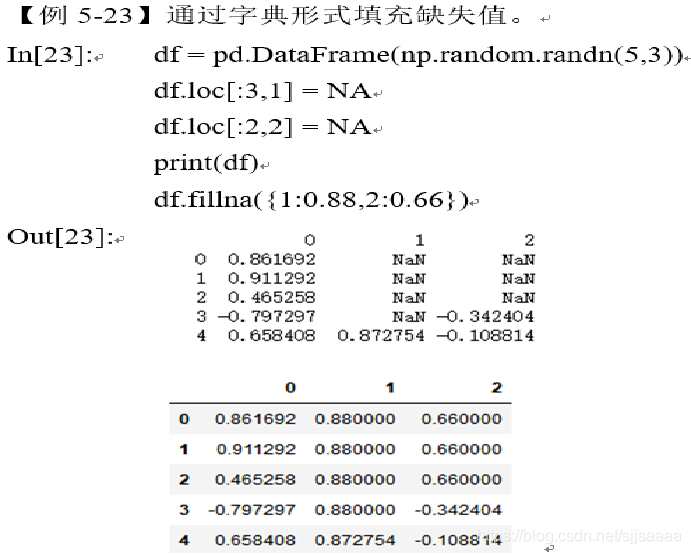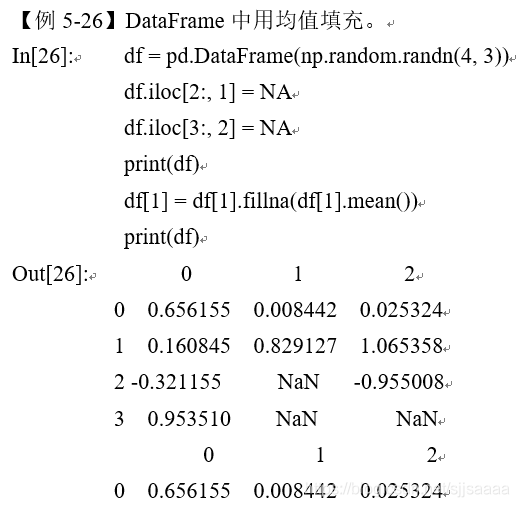Pandas通过drop_duplicates删除重复的行，格式为：
drop_duplicates(self, subset=None, keep=’first’, inplace=False)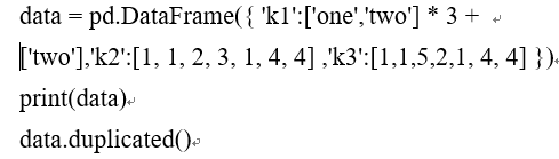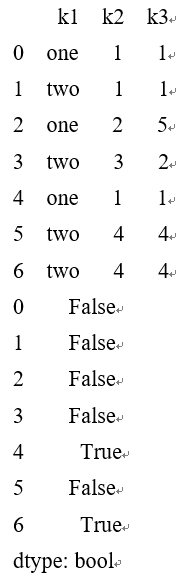drop_duplicates的主要参数及其说明：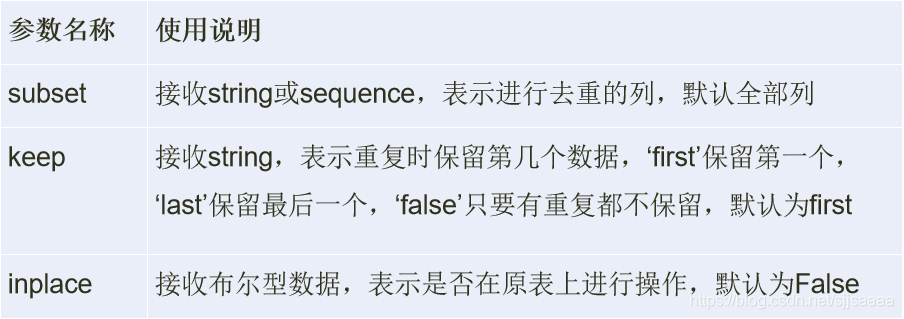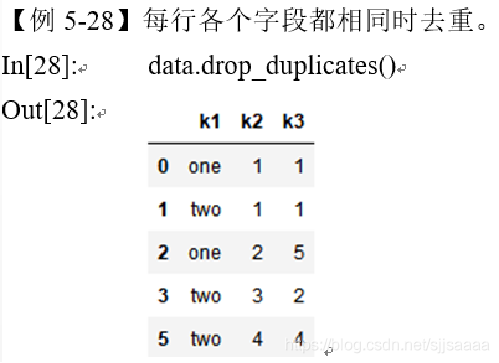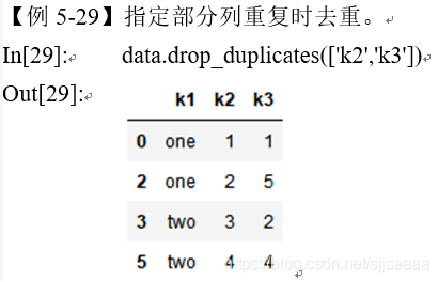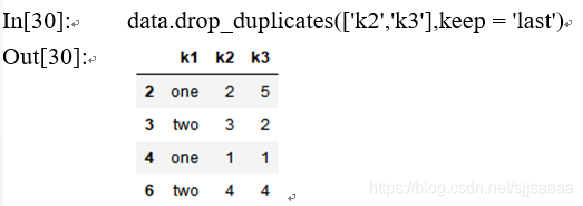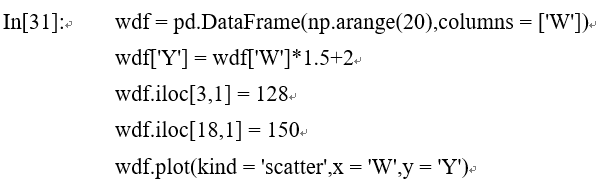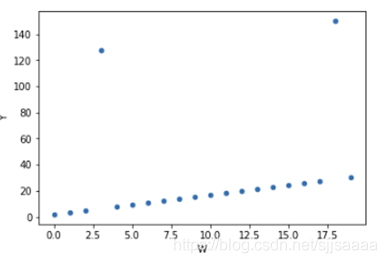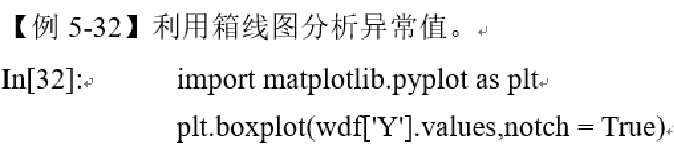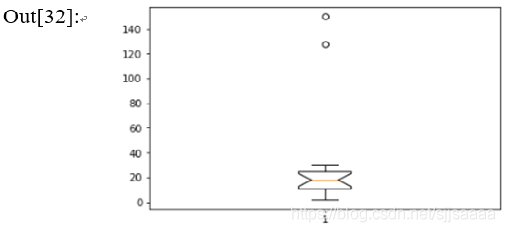3σ法则: 在3σ原则下，异常值被定义为一组测定值中与平均值的偏差超过3倍标准差的值。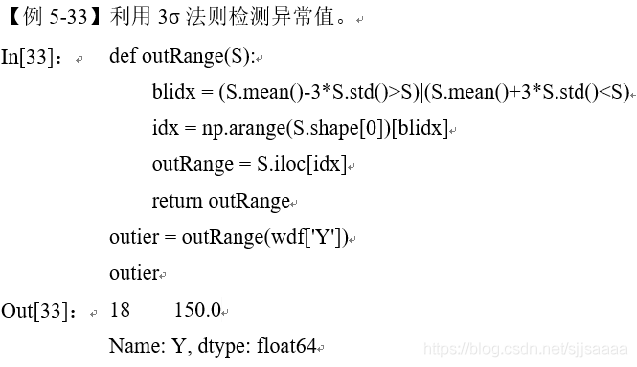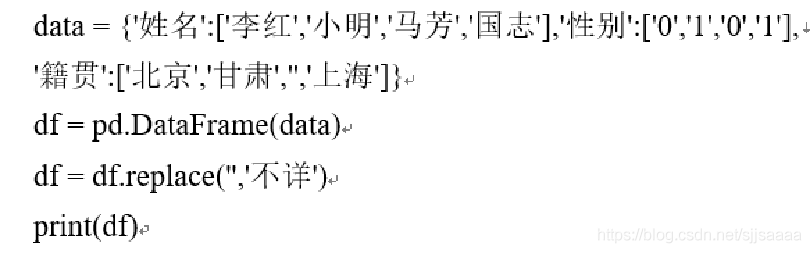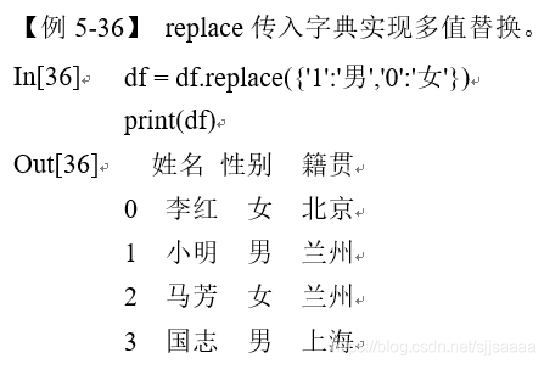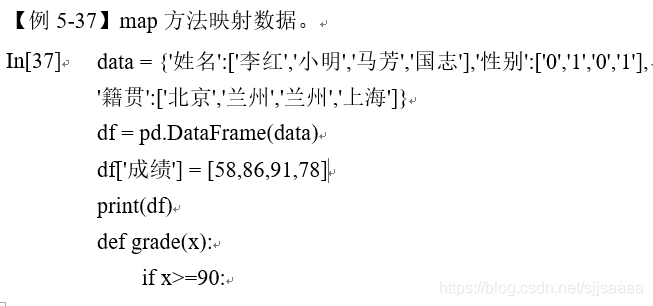## 4.数据标准化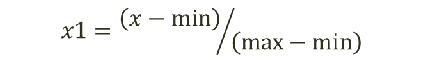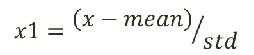## 5.数据转换

pandas.get_dummies(data, prefix = None, prefix_sep = '_', dummy_na = False, columns = None, sparse = False, drop_first=False)

get_dummies方法的主要参数及其说明：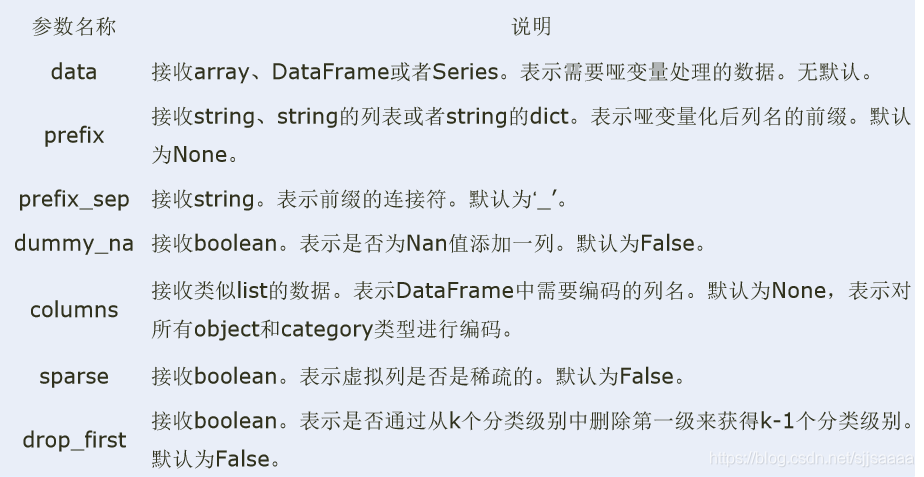1. 等宽法
Pandas提供了cut函数，可以进行连续型数据的等宽离散化。

pandas.cut(x, bins, right=True, labels=None, retbins=False, precision=3)

cut方法的主要参数及其说明：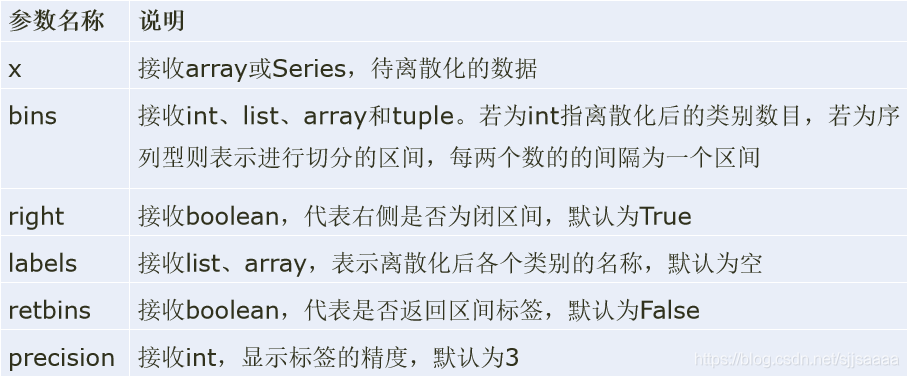1. 等频法
cut函数虽然不能够直接实现等频离散化，但可以通过定义将相同数量的记录放进每个区间。

2. 聚类分析法
一维聚类的方法包括两步，首先将连续型数据用聚类算法进行聚类，然后处理聚类得到的簇，为合并到一个簇的连续型数据做同一标记。